# Mathematics | Graph Theory Basics – Set 2

Prerequisite – Graph Theory Basics – Set 1
A graph is a structure amounting to a set of objects in which some pairs of the objects are in some sense “related”. The objects of the graph correspond to vertices and the relations between them correspond to edges. A graph is depicted diagrammatically as a set of dots depicting vertices connected by lines or curves depicting edges.
Formally,

“A graphconsists of, a non-empty set of vertices (or nodes) and, a set of edges. Each edge has either one or two vertices associated with it, called its endpoints.”

Types of graph :There are several types of graphs distinguished on the basis of edges, their direction, their weight etc.

1. Simple graph – A graph in which each edge connects two different vertices and where no two edges connect the same pair of vertices is called a simple graph. For example, Consider the following graph –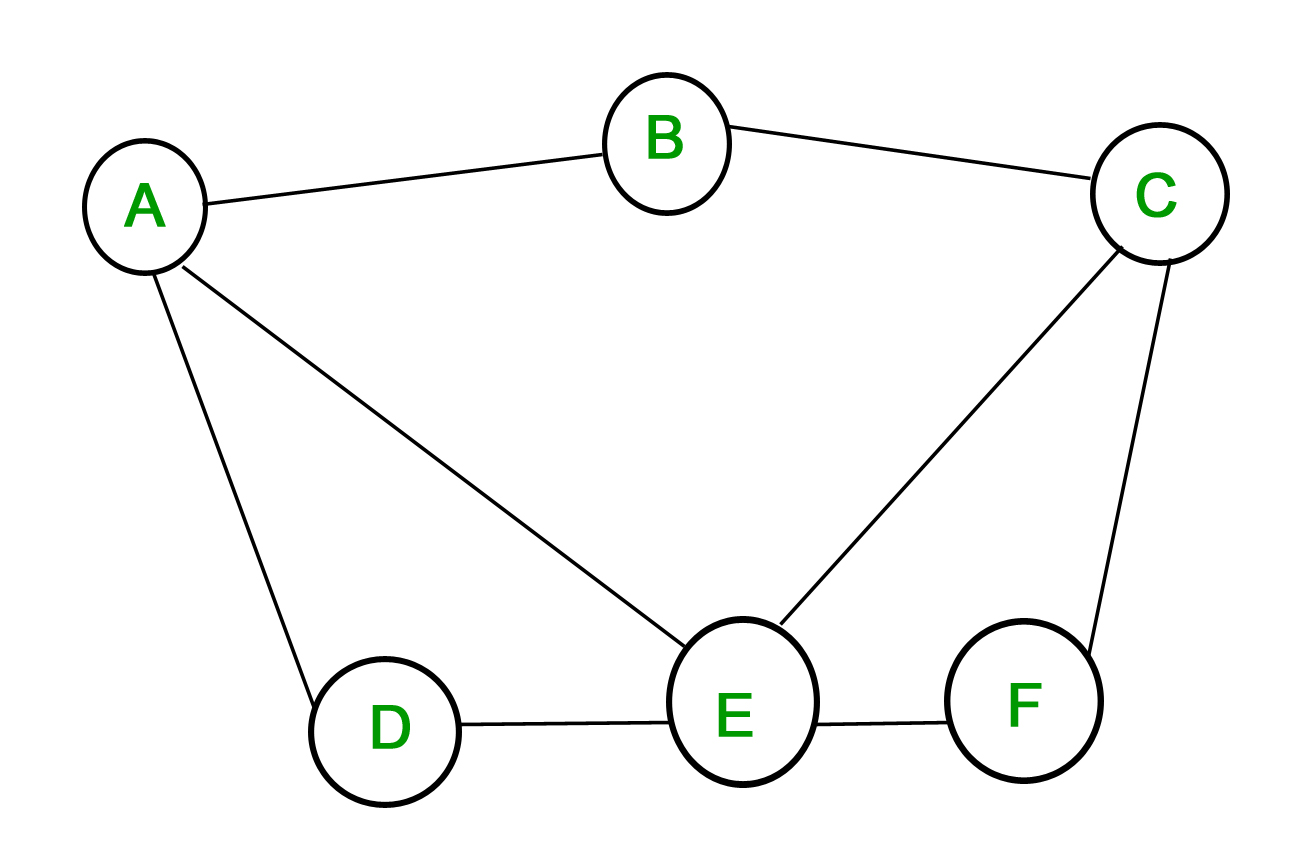The above graph is a simple graph, since no vertex has a self-loop and no two vertices have more than one edge connecting them.
The edges are denoted by the vertices that they connect-is the edge connecting verticesand.

2. Multigraph – A graph in which multiple edges may connect the same pair of vertices is called a multigraph.
Since there can be multiple edges between the same pair of vertices, the multiplicity of edge tells the number of edges between two vertices.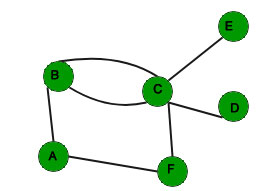The above graph is a multigraph since there are multiple edges betweenand. The multiplicity of the edgeis 2.

In some graphs, unlike the one’s shown above, the edges are directed. This means that the relation between the objects is one-way only and not two-way. The direction of the edges may be important in some applications.

Based on whether the edges are directed or not we can have directed graphs and undirected graphs. This property can be extended to simple graphs and multigraphs to get simple directed or undirected simple graphs and directed or undirected multigraphs.

Basic graph Terminology :

In the above discussion some terms regarding graphs have already been explained such as vertices, edges, directed and undirected edges etc. There are more terms which describe properties of vertices and edges.

• Adjacency – In a graphtwo verticesandare said to be adjacent if they are the endpoints of an edge. The edgeis said to be incident with the vertices.
In case the edge is directed,is said to be adjacent toandis said to be adjacent from. Here,is said to be the intitial vertex andis said to the terminal vertex.
• Degree – The degree of a vertex is the number of edges incident with it, except the self-loop which contributes twice to the degree of the vertex. Degree of a vertexis denoted as.
In case of directed graphs, the degree is further classified as in-degree and out-degree. The in-degree of a vertex is the number of edges with the given vertex as the terminal vertex. The out-degree of a vertex is the number of edges with the given vertex as the initial vertex. In-degree is denoted asand out-degree is denoted as.
For example in the directed graph shown above depicting flights between cities, the in-degree of the vertex “Delhi” is 3 and its out-degree is also 3.

Note: If a vertex has zero degree, it is called isolated. If the degree is one then it’s called pendant.

Handshaking Theorem :

What would one get if the degrees of all the vertices of a graph are added. In case of an undirected graph, each edge contributes twice, once for its initial vertex and second for its terminal vertex. So the sum of degrees is equal to twice the number of edges. This fact is stated in the Handshaking Theorem.

Letbe an undirected graph withedges. ThenIn case G is a directed graph,The handshaking theorem, for undirected graphs, has an interesting result –

An undirected graph has an even number of vertices of odd degree.


Proof : Letandbe the sets of vertices of even and odd degrees respectively.
We know by the handshaking theorem that,So,The sum of degrees of vertices with even degrees is even. The LHS is also even, which means that the sum of degrees of vertices with odd degrees must be even.
Thus, the number of vertices with odd degree is even.

Some special Simple Graphs :

1. Complete Graphs – A simple graph ofvertices having exactly one edge between each pair of vertices is called a complete graph. A complete graph ofvertices is denoted by. Total number of edges are n*(n-1)/2 with n vertices in complete graph.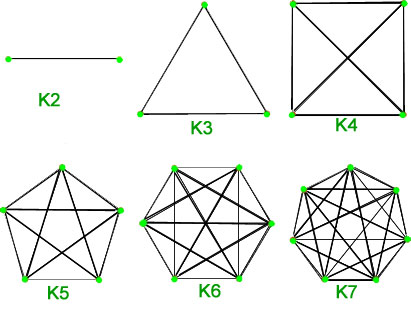2. Cycles – Cycles are simple graphs with verticesand edges. Cycle withvertices is denoted as. Total number of edges are n with n vertices in cycle graph.3. Wheels – A wheel is just like a cycle, with one additional vertex which is connected to every other vertex. Wheels ofvertices with 1 addition vertex are denoted by. Total number of edges are 2*(n-1) with n vertices in wheel graph.4. Hypercube – The Hypercube or n-cube is a graph withvertices each represented by a n-bit string. The vertices which differ by at most 1-bit are connected by edges. A hypercube ofvertices is denoted by. Total number of edges are n*withvertices in cube graph.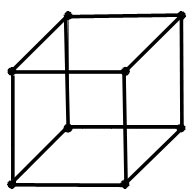5. Bipartite Graphs – A simple graphis said to be bipartite if its vertex setcan be divided into two disjoint sets such that every edge inhas its initial vertex in the first set and the terminal vertex in the second set. Total number of edges are (n*m) with (n+m) vertices in bipartite graph.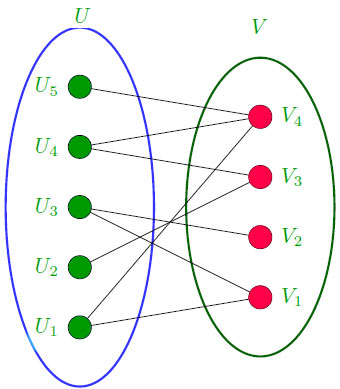Theorem – A simple graph is bipartite if and only if it is possible to assign one of two
different colors to each vertex of the graph so that no two adjacent are assigned the
same color.

A bipartite graph withandvertices in its two disjoint subsets is said to be complete if there is an edge from every vertex in the first set to every vertex in the second set, for a total ofedges. A complete bipartite graph withvertices in the first set andvertices in the second set is denoted as.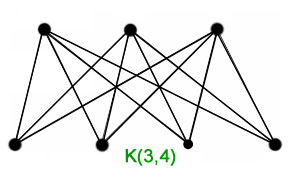GATE CS Corner Questions

Practicing the following questions will help you test your knowledge. All questions have been asked in GATE in previous years or in GATE Mock Tests. It is highly recommended that you practice them.

References-

Graphs – Wikipedia
Discrete Mathematics and its Applications, by Kenneth H Rosen

This article is contributed by Chirag Manwani. If you like GeeksforGeeks and would like to contribute, you can also write an article using contribute.geeksforgeeks.org or mail your article to contribute@geeksforgeeks.org. See your article appearing on the GeeksforGeeks main page and help other Geeks.

Attention reader! Don’t stop learning now. Get hold of all the important CS Theory concepts for SDE interviews with the CS Theory Course at a student-friendly price and become industry ready.

My Personal Notes arrow_drop_up

Article Tags :

10

Please write to us at contribute@geeksforgeeks.org to report any issue with the above content.